NEET  >  Test: Coulomb's Law (NCERT)

# Test: Coulomb's Law (NCERT)

Test Description

## 10 Questions MCQ Test Physics Class 12 | Test: Coulomb's Law (NCERT)

Test: Coulomb's Law (NCERT) for NEET 2023 is part of Physics Class 12 preparation. The Test: Coulomb's Law (NCERT) questions and answers have been prepared according to the NEET exam syllabus.The Test: Coulomb's Law (NCERT) MCQs are made for NEET 2023 Exam. Find important definitions, questions, notes, meanings, examples, exercises, MCQs and online tests for Test: Coulomb's Law (NCERT) below.
Solutions of Test: Coulomb's Law (NCERT) questions in English are available as part of our Physics Class 12 for NEET & Test: Coulomb's Law (NCERT) solutions in Hindi for Physics Class 12 course. Download more important topics, notes, lectures and mock test series for NEET Exam by signing up for free. Attempt Test: Coulomb's Law (NCERT) | 10 questions in 10 minutes | Mock test for NEET preparation | Free important questions MCQ to study Physics Class 12 for NEET Exam | Download free PDF with solutions
 1 Crore+ students have signed up on EduRev. Have you?
Test: Coulomb's Law (NCERT) - Question 1

### The constant k in Coulomb's law depends on

Detailed Solution for Test: Coulomb's Law (NCERT) - Question 1

The value of k = (1/4πε0) = 8.854 x 10-12 C2 N-1 m-2 where εis permittivity of free space.

Test: Coulomb's Law (NCERT) - Question 2

### Which of the following statement is not a similarity between electrostatic and gravitational forces?

Detailed Solution for Test: Coulomb's Law (NCERT) - Question 2

Electrostatic forces are both attractive and repulsive depending upon the type of charge, but gravitational forces is always attractive.

Test: Coulomb's Law (NCERT) - Question 3

### SI unit of permittivity of free space is

Test: Coulomb's Law (NCERT) - Question 4

The force between two small charged spheres having charges of 1 x 10-7 C and 2 x 10-7 C placed 20 cm apart in air is

Detailed Solution for Test: Coulomb's Law (NCERT) - Question 4

Here, q1 = 1 x 10-7 C, q2 = 2 x 10-7 C, r = 20cm = 20 x 10-2 m

Test: Coulomb's Law (NCERT) - Question 5

The nucleus of helium atom contains two proton that are separated by distance 3.0 x 10-15 m. The magnitude of the electrostatic force that each proton exerts on the other is

Detailed Solution for Test: Coulomb's Law (NCERT) - Question 5

Charge of proton is qP = 1.6 x 10-19 C

Distance between the protons is, r = 3 x 10-15m
The magnitude of electrostatic force between protons is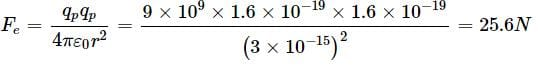Test: Coulomb's Law (NCERT) - Question 6

Two insulated charged metallic spheres P and Q have their centres separated by a distance of 60 cm. The radii of P and Q are negligible compared to the distance of separation. The mutual force of electrostatic repulsion if the charge on each is 3.2 x 10-7 C is

Detailed Solution for Test: Coulomb's Law (NCERT) - Question 6

Here, q1 = q2 = 3.2 x 10-7 C, r = 60cm = 0.6 m
Electrostatic force,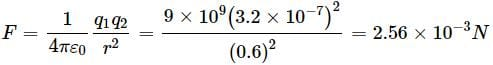Test: Coulomb's Law (NCERT) - Question 7

Two point charges of +3 μC and +4 μC repel each other with a force of 10 N. If each is given an additional charge of -6 μC, the new force is

Detailed Solution for Test: Coulomb's Law (NCERT) - Question 7

Here, q1 = +3 μC, q2 = +4 μC, F = 10N
q1 = +3 - 6 = -3 μC, q2 = +4 - 6 = -2 μC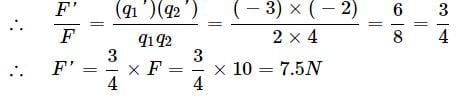Test: Coulomb's Law (NCERT) - Question 8

The ratio of magnitude of electrostatic force and gravitational force for an electron and proton is

Detailed Solution for Test: Coulomb's Law (NCERT) - Question 8

Here, for an electron and a proton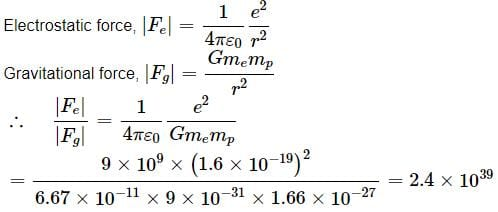Test: Coulomb's Law (NCERT) - Question 9

The electrostatic attracting force on a small sphere of charge 0.2μC due to another small sphere of charge -0.4μC in air is 0.4N. The distance between the two spheres is

Detailed Solution for Test: Coulomb's Law (NCERT) - Question 9

Here, q1 = 0.2 μC = 0.2 x 10-6 C
q2 = 0.4 μC = -0.4 x 10-6 C, F = -0.4 N
As F =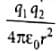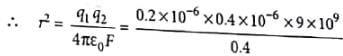= 1.8 x 10-3
∴ r = (1.8 x 10-3)1/2 = 0.0424 m = 42.4 x 10-3 m

Test: Coulomb's Law (NCERT) - Question 10

Under the action of a given coulombic force the acceleration of an electron is 2.5 x 1022 ms-2. Then the magnitude of the acceleration of a proton under the action of same force is nearly

Detailed Solution for Test: Coulomb's Law (NCERT) - Question 10

The acceleration due to given coulombic force F is
a = (F/m) or a ∝ (1/m) ...(1)
∴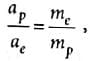where mc and mp are masses of electron and proton respectively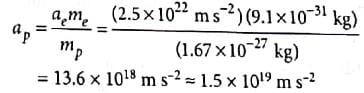## Physics Class 12

157 videos|452 docs|213 tests
 Use Code STAYHOME200 and get INR 200 additional OFF Use Coupon Code
Information about Test: Coulomb's Law (NCERT) Page
In this test you can find the Exam questions for Test: Coulomb's Law (NCERT) solved & explained in the simplest way possible. Besides giving Questions and answers for Test: Coulomb's Law (NCERT), EduRev gives you an ample number of Online tests for practice

## Physics Class 12

157 videos|452 docs|213 tests

### How to Prepare for NEET

Read our guide to prepare for NEET which is created by Toppers & the best Teachers